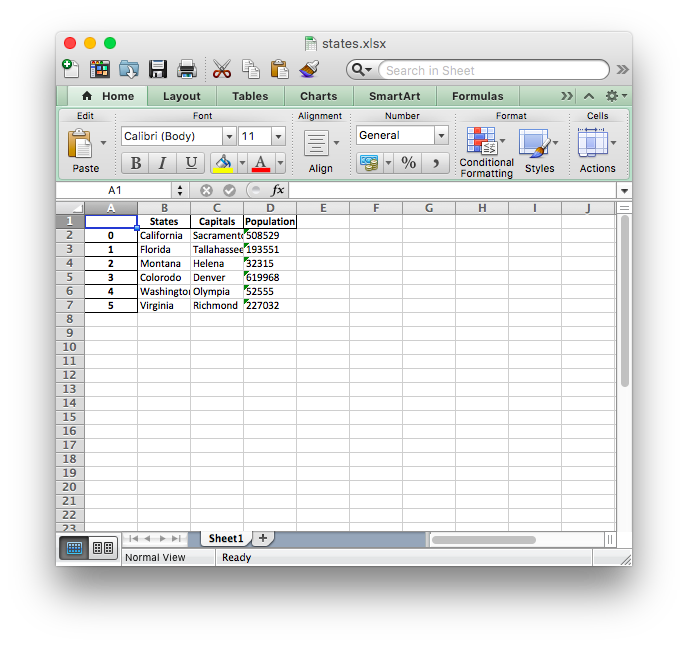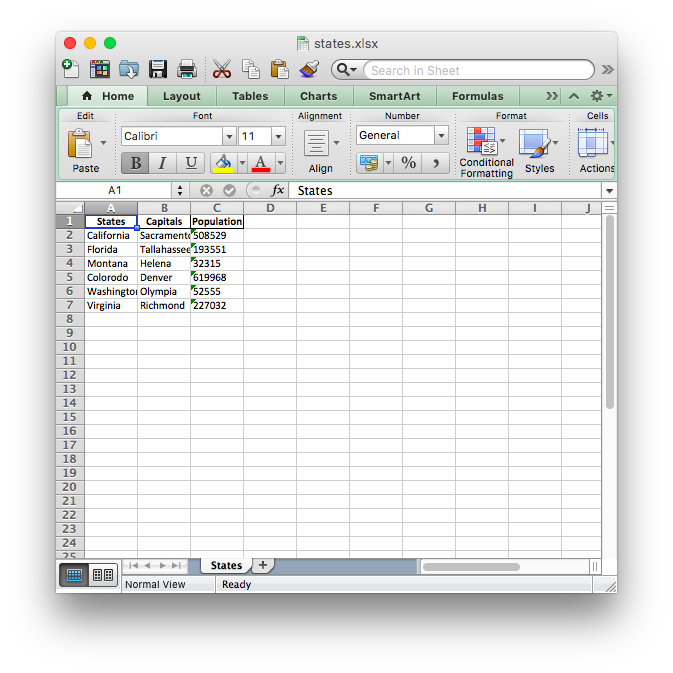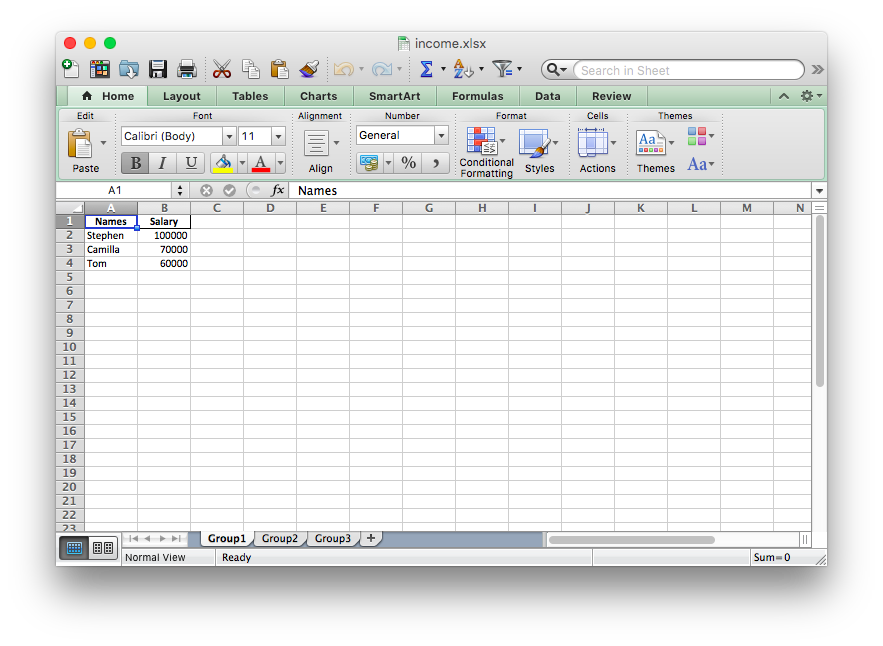## Reading and Writing Excel (XLSX) Files in Python with the Pandas Library

### Introduction

Just like with all other types of files, you can use the Pandas library to read and write Excel files using Python as well. In this short tutorial, we are going to discuss how to read and write Excel files via DataFrames.

In addition to simple reading and writing, we will also learn how to write multiple DataFrames into an Excel file, how to read specific rows and columns from a spreadsheet, and how to name single and multiple sheets within a file before doing anything.

### Reading and Writing Excel Files in Python with Pandas

Naturally, to use Pandas, we first have to install it. The easiest method to install it is via pip.

If you're running Windows:

$python pip install pandas  If you're using Linux or MacOS: $ pip install pandas


Note that you may get a ModuleNotFoundError or ImportError error when running the code in this article. For example:

ModuleNotFoundError: No module named 'openpyxl'


If this is the case, then you'll need to install the missing module(s):

\$ pip install openpyxl xlsxwriter xlrd


### Writing Excel Files Using Pandas

We'll be storing the information we'd like to write to an Excel file in a DataFrame. Using the built-in to_excel() function, we can extract this information into an Excel file.

First, let's import the Pandas module:

import pandas as pd


Now, let's use a dictionary to populate a DataFrame:

df = pd.DataFrame({'States':['California', 'Florida', 'Montana', 'Colorodo', 'Washington', 'Virginia'],
'Capitals':['Sacramento', 'Tallahassee', 'Helena', 'Denver', 'Olympia', 'Richmond'],
'Population':['508529', '193551', '32315', '619968', '52555', '227032']})


The keys in our dictionary will serve as column names. Similarly, the values become the rows containing the information.

Now, we can use the to_excel() function to write the contents to a file. The only argument is the file path:

df.to_excel('./states.xlsx')


Here's the Excel file that was created:Please note that we are not using any parameters in our example. Therefore, the sheet within the file retains its default name - "Sheet 1". As you can see, our Excel file has an additional column containing numbers. These numbers are the indices for each row, coming straight from the Pandas DataFrame.

We can change the name of our sheet by adding the sheet_name parameter to our to_excel() call:

df.to_excel('./states.xlsx', sheet_name='States')


Similarly, adding the index parameter and setting it to False will remove the index column from the output:

df.to_excel('./states.xlsx', sheet_name='States', index=False)



Now, the Excel file looks like this:#### Writing Multiple DataFrames to an Excel File

It is also possible to write multiple dataframes to an Excel file. If you'd like to, you can set a different sheet for each dataframe as well:

income1 = pd.DataFrame({'Names': ['Stephen', 'Camilla', 'Tom'],
'Salary':[100000, 70000, 60000]})

income2 = pd.DataFrame({'Names': ['Pete', 'April', 'Marty'],
'Salary':[120000, 110000, 50000]})

income3 = pd.DataFrame({'Names': ['Victor', 'Victoria', 'Jennifer'],
'Salary':[75000, 90000, 40000]})

income_sheets = {'Group1': income1, 'Group2': income2, 'Group3': income3}
writer = pd.ExcelWriter('./income.xlsx', engine='xlsxwriter')

for sheet_name in income_sheets.keys():
income_sheets[sheet_name].to_excel(writer, sheet_name=sheet_name, index=False)

writer.save()



Here, we've created 3 different dataframes containing various names of employees and their salaries as data. Each of these dataframes is populated by its respective dictionary.

We've combined these three within the income_sheets variable, where each key is the sheet name, and each value is the DataFrame object.

Finally, we've used the xlsxwriter engine to create a writer object. This object is passed to the to_excel() function call.

Before we even write anything, we loop through the keys of income and for each key, write the content to the respective sheet name.

Here is the generated file:You can see that the Excel file has three different sheets named Group1, Group2, and Group3. Each of these sheets contains names of employees and their salaries with respect to the date in the three different dataframes in our code.

The engine parameter in the to_excel() function is used to specify which underlying module is used by the Pandas library to create the Excel file. In our case, the xlsxwriter module is used as the engine for the ExcelWriter class. Different engines can be specified depending on their respective features.

Depending upon the Python modules installed on your system, the other options for the engine attribute are: openpyxl (for xlsx and xlsm), and xlwt (for xls).

Further details of using the xlsxwriter module with Pandas library are available at the official documentation.

Last but not least, in the code above we have to explicitly save the file using writer.save(), otherwise it won't be persisted on the disk.

### Reading Excel Files with Pandas

In contrast to writing DataFrame objects to an Excel file, we can do the opposite by reading Excel files into DataFrames. Packing the contents of an Excel file into a DataFrame is as easy as calling the read_excel() function:

students_grades = pd.read_excel('./grades.xlsx')



For this example, we're reading this Excel file.

Here, the only required argument is the path to the Excel file. The contents are read and packed into a DataFrame, which we can then preview via the head() function.

Note: Using this method, although the simplest one, will only read the first sheet.

Let's take a look at the output of the head() function:Pandas assigns a row label or numeric index to the DataFrame by default when we use the read_excel() function.

We can override the default index by passing one of the columns in Excel file column as the index_col parameter:

students_grades = pd.read_excel('./grades.xlsx', sheet_names='Grades', index_col='Grade')



Running this code will result in:In the example above, we have replaced the default index with the "Grade" column from the Excel file. However, you should only override the default index if you have a column with values that could serve as a better index.

#### Reading Specific Columns from an Excel File

Reading a file in its entirety is useful, though in many cases, you'd really want to access a certain element. For example, you might want to read the element's value and assign it to a field of an object.

Again, this is done using the read_excel() function, though, we'll be passing the usecols parameter. For example, we can limit the function to only read certain columns. Let's add the parameter so that we read the columns that correspond to the "Student Name", "Grade" and "Marks Obtained" values.

We do this by specifying the numeric index of each column:

cols = [0, 1, 3]



Running this code will yield:As you can see, we are only retrieving the columns specified in the cols list.

### Conclusion

We've covered some general usage of the read_excel() and to_excel() functions of the Pandas library. With them, we've read existing Excel files and written our own data to them.

Using various parameters, we can alter the behavior of these functions, allowing us to build customized files, rather than just dumping everything from a DataFrame.